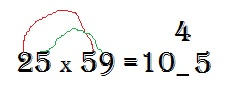## Friday, June 15, 2012

### The 3rd Graders Guide to Multiplying Two Digit Numbers

While I was recently preparing to take my GMAT I couldn't help but feel incredibly stupid trying to do math in my head and on paper. The fact is I’d become completely enslaved by my calculator. Only five years out of college and I could barely multiply and divide. That’s when I found this nice little tidbit of information to easily multiply two digit numbers by other two digit numbers.

How to multiply two digit numbers together in less than 6 seconds:Step 1: Find the first piece of the solution.

Multiply the first two digits in each number.

These are the first two digits of the solution.
Insert a blank after the result.Step 2: Find the last number of the solution.

Multiply the last two digits in the numbers of the problem. Then write the result after the blank. (Note that if the result is itself a two digit number we must carry a digit over the blank)Step 3: Fill in the blank.

This step can be the trickiest of the process (although it’s still simple).

First multiply the outside digits of the problem.

Then multiply the inside digits of the problem.
Add the results together and you have your number that goes in the blank.

Note that if the result is itself a two digit number we must carry a digit over a place, also if you had to carry a number over the blank then we add that to the solution as well.